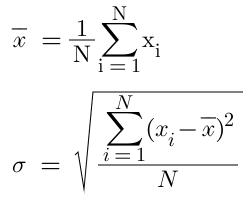Open in App
Not now

# Computing the Mean and Std of a Dataset in Pytorch

• Last Updated : 04 Jul, 2021

PyTorch provides various inbuilt mathematical utilities to monitor the descriptive statistics of a dataset at hand one of them being mean and standard deviation. Mean, denoted by, is one of the Measures of central tendencies which is calculated by finding the average of the given dataset. Standard Deviation, denoted by σ, is one of the measures of dispersion that signifies by how much are the values close to the mean. The formula for mean and standard deviation are as follows:-### Installing PyTorch:

Installing PyTorch is the same as that of any other library in python.

`pip install torch`

Or if you want to install it in a conda environment you can use the following command:-

`conda install pytorch cudatoolkit=10.2 -c pytorch`

### Mean and Standard Deviation of 1-D Tensor:

Before understanding how to find mean and standard deviation let’s ready our dataset by generating a random array.

```import torch
data = torch.rand(10)```

Now that we have the data we can find the mean and standard deviation by calling mean() and std() methods.

```mean_tensor = data.mean()
std_tensor = data.std()```

The above method works perfectly, but the values are returned as tensors, if you want to extract values inside that tensor you can either access it via index or you can call item() method.

```mean = data.mean().item()
std = data.std().item()```

Example:

## Python3

 `import` `torch`` ` `# Generate a tensor of 10 numbers``data ``=` `torch.rand(``10``)     `` ` `mean_tensor ``=` `data.mean()``std_tensor ``=` `data.std()`` ` `print``(mean_tensor)``print``(std_tensor)`` ` `mean ``=` `data.mean().item()``std ``=` `data.std().item()`` ` `print``(mean)``print``(std)`

Output:

```tensor(0.3901)
tensor(0.2846)
0.39005300402641296
0.2846093773841858```

### Mean and Standard Deviation of 2-D Tensors:

In 2-D Tensors mean is the same as that of the 1-D tensor except here we can pass an axis parameter to find the mean and std of the rows and columns. Let’s start by getting our data.

```import torch
data = torch.rand(5,3)```

The mean() and std() methods when called as is will return the total standard deviation of the whole dataset, but if we pass an axis parameter we can find the mean and std of rows and columns. For axis = 0, we get a tensor having values of mean or std of each column. For axis = 1, we get a tensor having values of mean or std of each row.

```total_mean = data.mean()
total_std = data.std()

# Mean and STD of columns
mean_col_wise = data.mean(axis = 0)
std_col_wise = data.std(axis = 0)

# Mean and STD of rows
mean_row_wise = data.mean(axis = 1)
std_row_wise = data.std(axis = 1)```

Example:

## Python3

 `import` `torch`` ` `# Generate a tensor of shape (5,3)``data ``=` `torch.rand(``5``,``3``)      `` ` `total_mean ``=` `data.mean()``total_std ``=` `data.std()`` ` `print``(total_mean)``print``(total_std)`` ` `# Mean and STD of columns``mean_col_wise ``=` `data.mean(axis ``=` `0``)``std_col_wise ``=` `data.std(axis ``=` `0``)`` ` `print``(mean_col_wise)``print``(std_col_wise)`` ` `# Mean and STD of rows``mean_row_wise ``=` `data.mean(axis ``=` `1``)``std_row_wise ``=` `data.std(axis ``=` `1``)`` ` `print``(mean_row_wise)``print``(std_row_wise)`

Output:

```tensor(0.6483)
tensor(0.2797)
tensor([0.6783, 0.5986, 0.6679])
tensor([0.2548, 0.2711, 0.3614])
tensor([0.5315, 0.7770, 0.7785, 0.3403, 0.8142])
tensor([0.3749, 0.2340, 0.1397, 0.2432, 0.1386])```

My Personal Notes arrow_drop_up